/

### The Student’s t distribution

The Student’s t distribution (more simply the t distribution) arises when estimating the mean of a normally distributed population when sample sizes are small and the population standard deviation is unknown.

 Distribution name (Standard) Student’s t distribution Common notation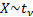Parameters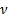= degrees of freedom (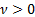, usuallyis an integer although in some situations a non-integralcan arise) Domain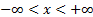Probability density function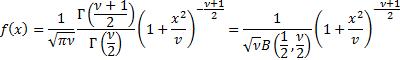Cumulative distribution function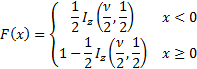where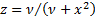Mean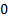Variance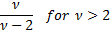Skewness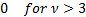(Excess) kurtosis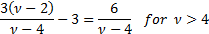Characteristic function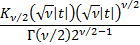where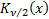is a Bessel function Other comments It is a special case of the generalised hyperbolic distribution.   Its non-central moments ifis even and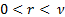are: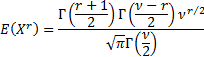Ifis even and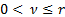then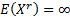, ifis odd andthen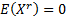and ifis odd andthen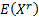is undefined.

Nematrian web functions

Functions relating to the above distribution may be accessed via the Nematrian web function library by using a DistributionName of “student's t”. Functions relating to a generalised version of this distribution including additional location (i.e. shift) and scale parameters may be accessed by using a DistributionName of “student's t3”, see also including additional shift and scale parameters. For details of other supported probability distributions see here.